Image Transformation

Besides representing images in the spatial domain (i.e. the Cartesian coordinates), images can also be represented in many other domains. The one that is used most often in image processing is the frequency domain. In order for an image to be represented in the frequency domain, one or more transformation must be performed. Readers have probably seen the 1-Dimensional Fourier transform in a signals and systems course. The 2-Dimensional Fourier transform can be applied to images to obtain their frequency representations. The 2-D Fourier transform is actually an extension to the 1-D case. The transform is given mathematically by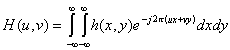and the inverse 2-D Fourier transform is given by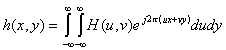The discrete Fourier transform (DFT) is a special case of the Fourier transform. It is needed when working with digital images since digital images have a finite number of discrete samples. A digital image is usually obtained from sampling the continuous image. The image pixels are then normally truncated to 8-12 bits per pixel before being processed or displayed. The formula for computing the discrete Fourier transform on an MxN size image is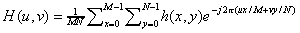and the formula for the inverse discrete Fourier transform is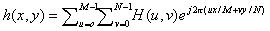4.1 Fast Fourier Transforms

The discrete Fourier transform is a computational intensive operation. It requires (MxN)2 complex multiplications. Fortunately, the computational complexity can be reduced to O(Nlog2N) by using the fast Fourier transform (FFT), which is a fast implementation of the discrete Fourier transform. Listing 4.1 shows the code of a 2-D FFT implementation. The program fft2 reads in an image, performs 2-D FFT or inverse 2-D FFT by calling the 1-D FFT routine for each row and column, and writes the resulting transform to the output file.

NAME
fft2 - performs 2D-FFT of an input image
SYNOPSIS
fft2(input_file_name)
fft2(input_file_name, [mrows, ncols])
fft2(input_file_name, mrows, ncols)
 mrows --pads or crops the image to mrowsXncols before transforming ncols --pads or crops the image to mrowsXncols before transforming

DESCRIPTION
fft2 performs 2D-FFT of an input image. It reads an image, crops the image if necessary before transforming, and performs the transformation. The output of the fft2 function is the frequency transform of the source image in complex data structures. The Fourier transform of the image is returned to the user and the magnitude of the Fourier transform is displayed on screen.
This implementation of fft2 makes use of the "fft" function provided by MATLAB. fft is first applied to the rows of the image; after that, fft is again applied to the columns of the row-transformed image.
EXAMPLES
lena_fft2=fft2('LENA.TIF')
This example performs 2D-FFT of LENA (Figure 5.1a) and stores the frequency transform of the image in the variable "lena_fft2".
ptr_fft2=fft2('PAINTER.TIF')
This example performs 2D-FFT of PAINTER (Figure 5.1b) and stores the frequency transform of the image in the variable "ptr_fft2".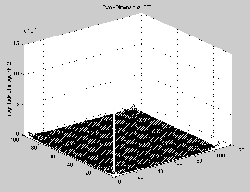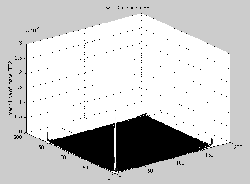a. Magnitude spectrum of LENA b. Magnitude spectrum of PAINTER

Figure 5.1 Examples of 2D-FFT transformed images

NAME
ifft2 - performs inverse 2D-FFT of a Fourier transformed image
SYNOPSIS
ifft2(x, output_file_name)
ifft2(x, output_file_name, [mrows, ncols])
ifft2(x, output_file_name, mrows, ncols)
 x --the frequency transformed image represented by a matrix mrows --pads or crops the image to mrowsXncols before inverse transformation ncols --pads or crops the image to mrowsXncols before inverse transformation

DESCRIPTION
ifft2 performs 2D-IFFT on a Fourier transformed input image. x, which is a complex matrix, is the Fouriery-transformed image. ifft2 reads in the image matrix, crops the image if necessary before transformation, and performs the inverse transformation. The output of the ifft2 function is written to the output file. The format of the output image is TIFF.
This implementation of ifft2 makes use of the "ifft" function provided by MATLAB. ifft is first applied to the rows of the image; after that, ifft is again applied to the columns of the row-transformed image.
EXAMPLES
ifft2(lena_fft2, 'if_lena.tif')
This example performs 2D-IFFT of the previously 2D-FFT transformed LENA (Figure 5.2a).
ifft2(ptr_fft2, 'if_ptr.tif')
This example performs 2D-IFFT of the previously 2D-FFT transformed PAINTER (Figure 5.2b)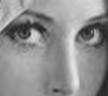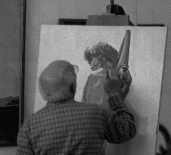a. Restored LENA via 2D-FFT b. Restored PAINTER via 2D-FFT

Figure 5.2 Examples of inverse 2D-FFT transformed images

Fourier Transform

Brief DescriptionThe Fourier Transform is an important image processing tool which is used to decompose an image into its sine and cosine components. The output of the transformation represents the image in the Fourier or frequency domain, while the input image is the spatial domain equivalent. In the Fourier domain image, each point represents a particular frequency contained in the spatial domain image.
The Fourier Transform is used in a wide range of applications, such as image analysis, image filtering, image reconstruction and image compression.How It Works

As we are only concerned with digital images, we will restrict this discussion to the Discrete Fourier Transform (DFT).
The DFT is the sampled Fourier Transform and therefore does not contain all frequencies forming an image, but only a set of samples which is large enough to fully describe the spatial domain image. The number of frequencies corresponds to the number of pixels in the spatial domain image, i.e. the image in the spatial and Fourier domain are of the same size.
For a square image of size N×N, the two-dimensional DFT is given by: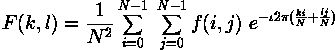where f(i,j) is the image in the spatial domain and the exponential term is the basis function corresponding to each point F(k,l) in the Fourier space. The equation can be interpreted as: the value of each point F(k,l) is obtained by multiplying the spatial image with the corresponding base function and summing the result.
The basis functions are sine and cosine waves with increasing frequencies, i.e. F(0,0) represents the DC-component of the image which corresponds to the average brightness and F(N-1,N-1) represents the highest frequency.
In a similar way, the Fourier image can be re-transformed to the spatial domain.The inverse Fourier transform is given by: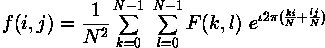To obtain the result for the above equations, a double sum has to be calculated for each image point. However, because the Fourier Transform is separable, it can be written aswhere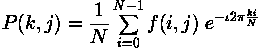Using these two formulas, the spatial domain image is first transformed into an intermediate image using N one-dimensional Fourier Transforms. This intermediate image is then transformed into the final image, again using N one-dimensional Fourier Transforms. Expressing the two-dimensional Fourier Transform in terms of a series of 2N one-dimensional transforms decreases the number of required computations.Even with these computational savings, the ordinary one-dimensional DFT has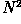complexity. This can be reduced to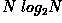if we employ the Fast Fourier Transform (FFT) to compute the one-dimensional DFTs. This is a significant improvement, in particular for large images. There are various forms of the FFT and most of them restrict the size of the input image that may be transformed, often to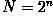where n is an integer. The mathematical details are well described in the literature.
The Fourier Transform produces a complex number valued output image which can be displayed with two images, either with the real and imaginary part or with magnitude and phase. In image processing, often only the magnitude of the Fourier Transform is displayed, as it contains most of the information of the geometric structure of the spatial domain image. However, if we want to re-transform the Fourier image into the correct spatial domain after some processing in the frequency domain, we must make sure to preserve both magnitude and phase of the Fourier image.
The Fourier domain image has a much greater range than the image in the spatial domain. Hence, to be sufficiently accurate, its values are usually calculated and stored in float values.Guidelines for Use

The Fourier Transform is used if we want to access the geometric characteristics of a spatial domain image. Because the image in the Fourier domain is decomposed into its sinusoidal components, it is easy to examine or process certain frequencies of the image, thus influencing the geometric structure in the spatial domain.
In most implementations the Fourier image is shifted in such a way that the DC-value (i.e. the image mean) F(0,0) is displayed in the center of the image. The further away from the center an image point is, the higher is its corresponding frequency.
We start off by applying the Fourier Transform ofThe magnitude calculated from the complex result is shown in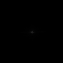We can see that the DC-value is by far the largest component of the image. However, the dynamic range of the Fourier coefficients (i.e. the intensity values in the Fourier image) is too large to be displayed on the screen, therefore all other values appear as black. If we apply a logarithmic transformation to the image we obtain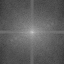The result shows that the image contains components of all frequencies, but that their magnitude gets smaller for higher frequencies. Hence, low frequencies contain more image information than the higher ones. The transform image also tells us that there are two dominating directions in the Fourier image, one passing vertically and one horizontally through the center. These originate from the regular patterns in the background of the original image.
The phase of the Fourier transform of the same image is shown in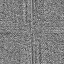The value of each point determines the phase of the corresponding frequency. As in the magnitude image, we can identify the vertical and horizontal lines corresponding to the patterns in the original image. The phase image does not yield much new information about the structure of the spatial domain image; therefore, in the following examples, we will restrict ourselves to displaying only the magnitude of the Fourier Transform.
Before we leave the phase image entirely, however, note that if we apply the inverse Fourier Transform to the above magnitude image while ignoring the phase (and then histogram equalize the output) we obtain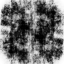Although this image contains the same frequencies (and amount of frequencies) as the original input image, it is corrupted beyond recognition. This shows that the phase information is crucial to reconstruct the correct image in the spatial domain.
We will now experiment with some simple images to better understand the nature of the transform. The response of the Fourier Transform to periodic patterns in the spatial domain images can be seen very easily in the following artificial images.
The imageshows 2 pixel wide vertical stripes. The Fourier transform of this image is shown in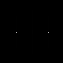If we look carefully, we can see that it contains 3 main values: the DC-value and, since the Fourier image is symmetrical to its center, two points corresponding to the frequency of the stripes in the original image. Note that the two points lie on a horizontal line through the image center, because the image intensity in the spatial domain changes the most if we go along it horizontally.
The distance of the points to the center can be explained as follows: the maximum frequency which can be represented in the spatial domain are one pixel wide stripes.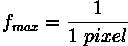Hence, the two pixel wide stripes in the above image represent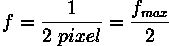Thus, the points in the Fourier image are halfway between the center and the edge of the image, i.e. the represented frequency is half of the maximum.
Further investigation of the Fourier image shows that the magnitude of other frequencies in the image is less than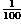of the DC-value, i.e. they don't make any significant contribution to the image. The magnitudes of the two minor points are each two-thirds of the DC-value.
Similar effects as in the above example can be seen when applying the Fourier Transform to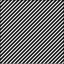which consists of diagonal stripes. In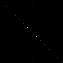showing the magnitude of the Fourier Transform, we can see that, again, the main components of the transformed image are the DC-value and the two points corresponding to the frequency of the stripes. However, the logarithmic transform of the Fourier Transform,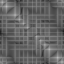shows that now the image contains many minor frequencies. The main reason is that a diagonal can only be approximated by the square pixels of the image, hence, additional frequencies are needed to compose the image. The logarithmic scaling makes it difficult to tell the influence of single frequencies in the original image. To find the most important frequencies we threshold the original Fourier image at level 13. The resulting Fourier image,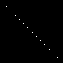shows all frequencies whose magnitude is at least 5% of the main peak. Compared to the original Fourier image, several more points appear. They are all on the same diagonal as the three main components, i.e. they all originate from the periodic stripes. The represented frequencies are all multiples of the basic frequency of the stripes in the spatial domain image. This is because a rectangular signal, like the stripes, with the frequency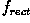is a composition of sine waves with the frequencies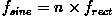, known as the harmonics of. All other frequencies disappeared from the Fourier image, i.e. the magnitude of each of them is less than 5% of the DC-value.A Fourier-Transformed image can be used for frequency filtering. A simple example is illustrated with the above image. If we multiply the (complex) Fourier image obtained above with an image containing a circle (of r = 32 pixels), we can set all frequencies larger thanto zero as shown in the logarithmic transformed image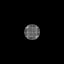By applying the inverse Fourier Transform we obtain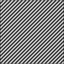The resulting image is a lowpass filtered version of the original spatial domain image. Since all other frequencies have been suppressed, this result is the sum of the constant DC-value and a sine-wave with the frequency. Further examples can be seen in the worksheet on frequency filtering.
A property of the Fourier Transform which is used, for example, for the removal of additive noise, is its distributivity over addition. We can illustrate this by adding the complex Fourier images of the two previous example images. To display the result and emphasize the main peaks, we threshold the magnitude of the complex image, as can be seen in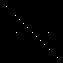Applying the inverse Fourier Transform to the complex image yields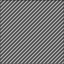According to the distributivity law, this image is the same as the direct sum of the two original spatial domain images.
Finally, we present an example (i.e. text orientation finding) where the Fourier Transform is used to gain information about the geometric structure of the spatial domain image. Text recognition using image processing techniques is simplified if we can assume that the text lines are in a predefined direction. Here we show how the Fourier Transform can be used to find the initial orientation of the text and then a rotation can be applied to correct the error. We illustrate this technique using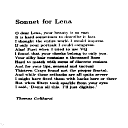a binary image of English text. The logarithm of the magnitude of its Fourier transform is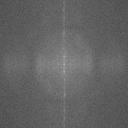and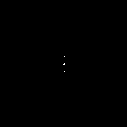is the thresholded magnitude of the Fourier image. We can see that the main values lie on a vertical line, indicating that the text lines in the input image are horizontal. If we proceed in the same way with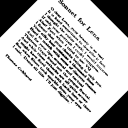which was rotated about 45°, we obtain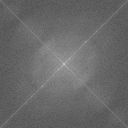and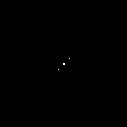in the Fourier space. We can see that the line of the main peaks in the Fourier domain is rotated according to rotation of the input image. The second line in the logarithmic image (perpendicular to the main direction) originates from the black corners in the rotated image.
Although we managed to find a threshold which separates the main peaks from the background, we have a reasonable amount of noise in the Fourier image resulting from the irregular pattern of the letters. We could decrease these background values and therefore increase the difference to the main peaks if we were able to form solid blocks out of the text-lines. This could, for example, be done by using a morphological operator.

Common VariantsAnother sinusoidal transform (i.e. transform with sinusoidal base functions) related to the DFT is the Discrete Cosine Transform (DCT). For an N×N image, the DCT is given by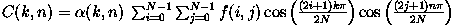with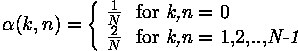The main advantages of the DCT are that it yields a real valued output image and that it is a fast transform. A major use of the DCT is in image compression --- i.e. trying to reduce the amount of data needed to store an image. After performing a DCT it is possible to throw away the coefficients that encode high frequency components that the human eye is not very sensitive to. Thus the amount of data can be reduced, without seriously affecting the way an image looks to the human eye.

Exercises

1. Take the Fourier Transforms ofandand add them using blend. Take the inverse Fourier Transform of the sum. Explain the result.

2. Using a paint program, create an image made of periodical patterns of varying frequency and orientation. Examine its Fourier Transform and investigate the effects of removing or changing some of the patterns in the spatial domain image.

3. Apply the mean operator toand compare its Fourier Transform before and after the operation.

4. Add different sorts of noise toand compare the Fourier Transforms with5. Use the open operator to transform the text lines in the above images into solid blocks. Make sure that the chosen structuring element works for all orientations of text. Compare the Fourier Transforms of the resulting images with the transforms of the unprocessed text images.

6. Investigate if the Fourier Transform is distributive over multiplication. To do so, multiplywithand take the Fourier Transform. Compare the result with the multiplication of the two direct Fourier Transforms.

References

D. Ballard and C. Brown Computer Vision, Prentice-Hall, 1982, pp 24 - 30.
R. Gonzales, R. Woods Digital Image Processing, Addison-Wesley Publishing Company, 1992, pp 81 - 125.
B. Horn Robot Vision, MIT Press, 1986, Chaps 6, 7.
A. Jain Fundamentals of Digital Image Processing, Prentice-Hall, 1989, pp 15 - 20.
A. Marion An Introduction to Image Processing, Chapman and Hall, 1991, Chap. 9.